Courses

# Test: Shapes And Space - 3

## 20 Questions MCQ Test Mathematics for Class 1: NCERT | Test: Shapes And Space - 3

Description
This mock test of Test: Shapes And Space - 3 for Class 1 helps you for every Class 1 entrance exam. This contains 20 Multiple Choice Questions for Class 1 Test: Shapes And Space - 3 (mcq) to study with solutions a complete question bank. The solved questions answers in this Test: Shapes And Space - 3 quiz give you a good mix of easy questions and tough questions. Class 1 students definitely take this Test: Shapes And Space - 3 exercise for a better result in the exam. You can find other Test: Shapes And Space - 3 extra questions, long questions & short questions for Class 1 on EduRev as well by searching above.
QUESTION: 1

Solution:
QUESTION: 2

Solution:
QUESTION: 3

### What is the shape of the picture given here?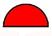Solution:
QUESTION: 4

Match the shape to its name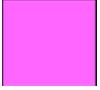Solution:

This is a square in shape.

QUESTION: 5

Which of the following is a square?

Solution:

(A)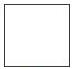this is a square

QUESTION: 6

Find the circle

Solution: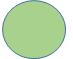This is a circle

QUESTION: 7

Which of the following the shape of disc?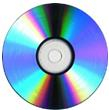Solution:
QUESTION: 8

Count the semi circles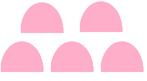Solution:
QUESTION: 9

Which of the following shapes can be found in the picture?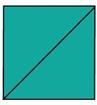Solution:
QUESTION: 10

Match the shape to its name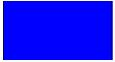Solution:
QUESTION: 11

Which of the following is a rectangle?

Solution: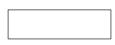This is a Rectangle in shape.

QUESTION: 12

Rearrange the letters to make a shape : BUEC

Solution:
QUESTION: 13

Which of the following is cone?

Solution:
QUESTION: 14

Find the Cone

Solution:
QUESTION: 15

Which of the following is a circle?

Solution:
QUESTION: 16

Match the shape to its name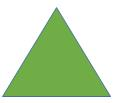Solution:
QUESTION: 17

What is the shape of the dice? Identify from the options below.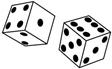Solution:

The dice are cube in shape.

QUESTION: 18

Find the Diamond

Solution:
QUESTION: 19

How many square are seen here?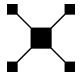Solution:
QUESTION: 20

Count the squares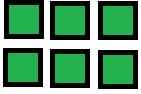Solution: